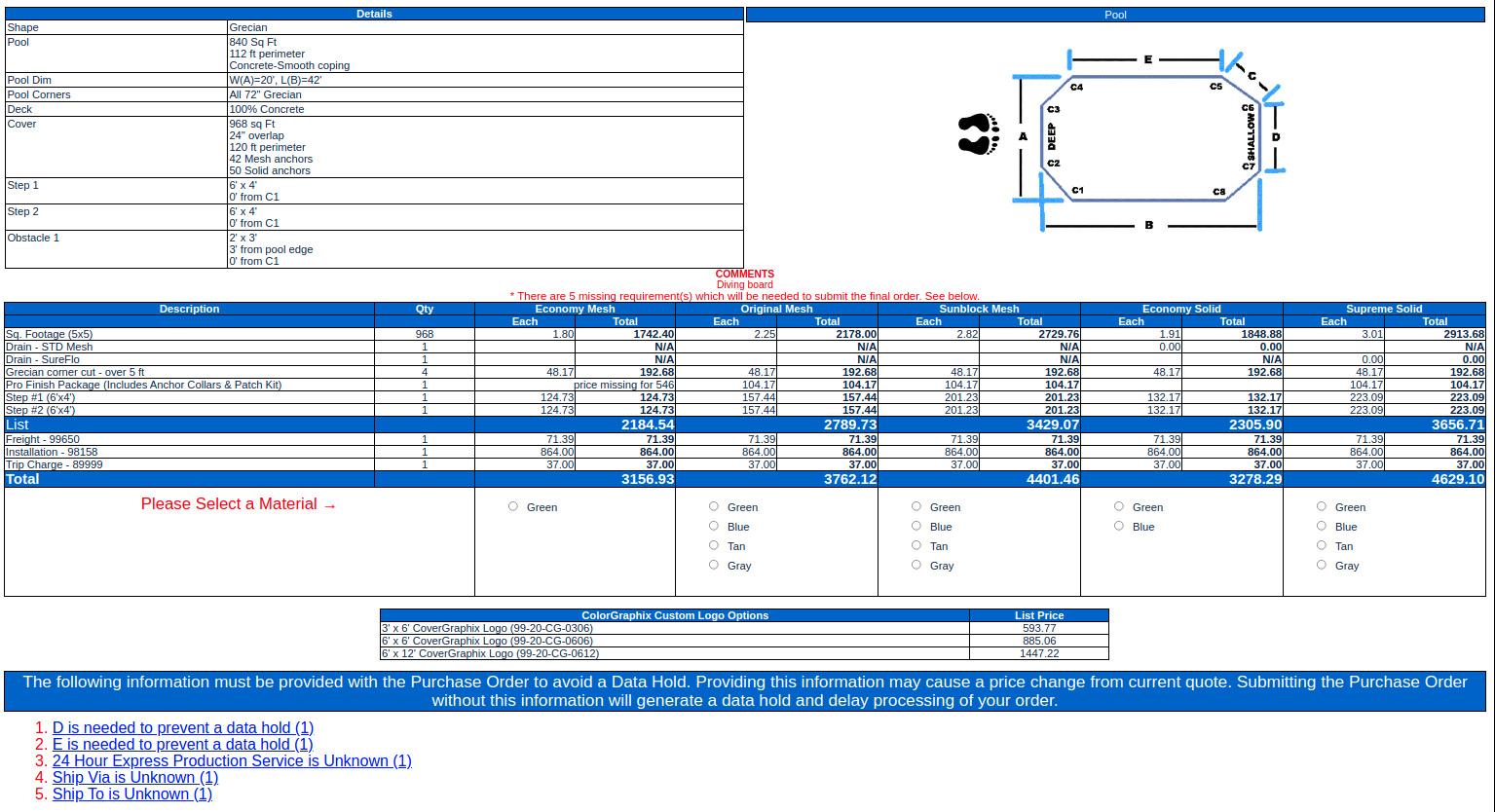# Pool Cover & Liner Estimator

Published on

## Project Specifications

Build a pool and liner quoting system to:

• Remove the bottleneck whereby only a small group of experienced estimators build quotes.
• Identify and pass easy quotes to the less experienced estimators, allowing the company to expand production.
• Reduce costly mistakes in misquoting and building covers.
• Automate pricing and custom logic rules.

## Solutions

• Hierarchical Customer Structure - I used a hierarchical customer structure which allowed customers to be the parent of other customers. So Distributors could have dealers under them. Distributor-groups could have distributors under them. Dealers, distributors, and distributor-groups all inherit pricing and custom rules from each other and can be overridden.
• Pricing - Multiple pricing levels could be created. Distributors could be assigned a pricing level and dealers would automatically inherit that pricing level. However, the dealer pricing level could be overridden. The arcitecture worked beautifully and was fast as lightining, using an SQL union and procedure to quickly calculate final pricing.
• Customer Logic Rules - Rules were simply products which therefore shared the logic from the pricing. This also gave an easy interface for handling rules. Implementation was fast as lightning and intuitive.

This PHP and MySQL LAMP application was integrated with NetSuite and involved building some rather complex solutions.

## Pool covers

1. Based on the Shape
2. Measure the Pool
3. Calculate the price

Sounds easy, right? Actual implementation was smooth and easy to use, with feedback helping the estimator with tips and warnings.## AB Covers

So, how do you measure a completely custom shape? You lay down two points near the pool and start measuring each point along the edge of the pool to that set of points.

A little math and now you have a polygon. Right? So how do you add 18" to the edge of that polygon/pool?

It's crazy but it can be done. I'll give you a hint, use vectors! For \mathbf{n} points which lay on the parimeter of a pool:

\mathbf{Points} = \left[\begin{array} {c} p_1 \\ . \\ . \\ . \\ p_n \end{array}\right]

we calculate a set of vectors between the points

\mathbf{Vectors} = \left[\begin{array} {c} v_1 (p_2 - p_1)\\ . \\ . \\ . \\ v_n (p_1 - p_n) \end{array}\right]

We then walk around the polygon calculating the correct Offset:

\mathbf{Cover} = \left[\begin{array} {c} v_1 + Offset \\ . \\ . \\ . \\ v_n + Offset \end{array}\right]

Which generates a polygon with an overlap of exactly 16", or whatever the customer ordered.Or to save time use a matrix:

\mathbf{P} = \left[\begin{array} {ccccc} p_{1} & . & . & . & p_{n} \\ . & . & . & . & . \\ . & . & . & . & . \\ . & . & . & . & . \\ p_{v1} & . & . & . & p_{vn} \end{array}\right] = \left[\begin{array} {c} \mathbf{x}'_pn \\ . \\ . \\ . \\ \mathbf{x}'_vn \end{array}\right]

There we have \mathbf{P} for drawing the new cover. Fun right?

## Liners

Liners are essentially the same thing but with three dimensions, instead of two.## Bottom Line

Our client was able to:

• Use experienced estimators more effectively